Publicité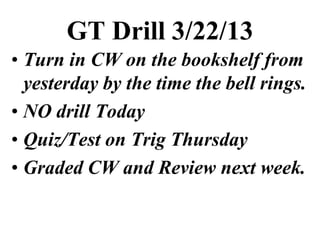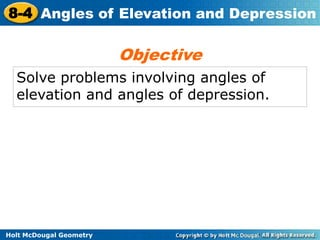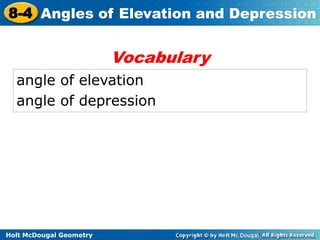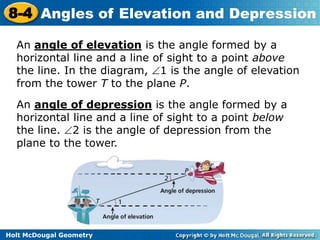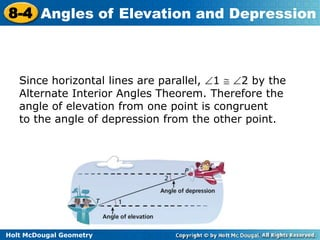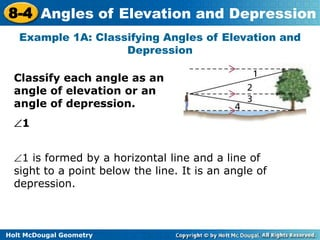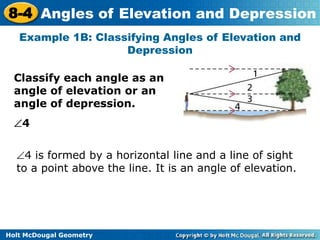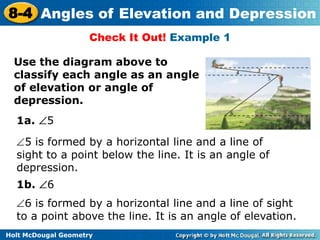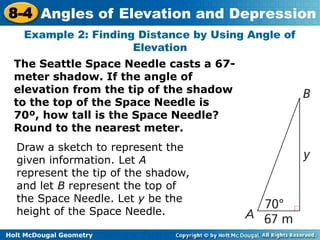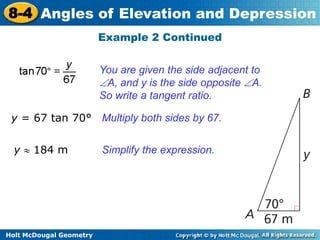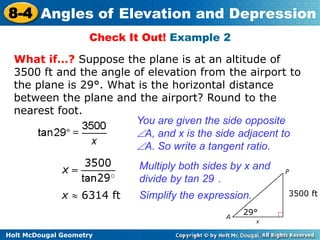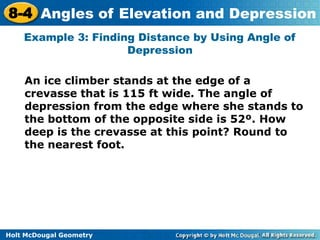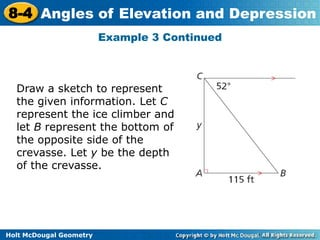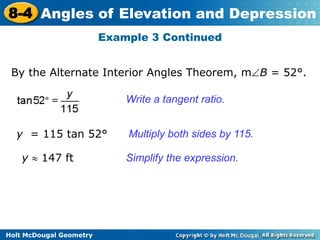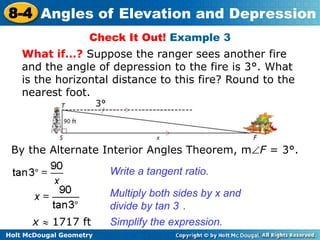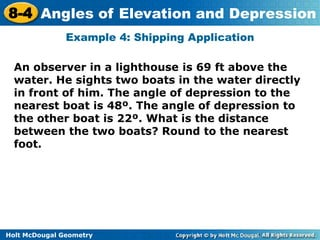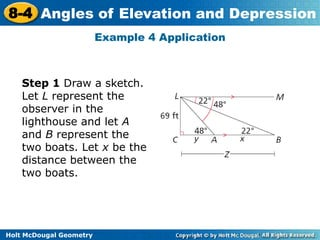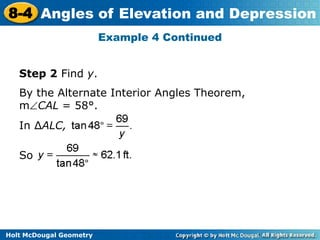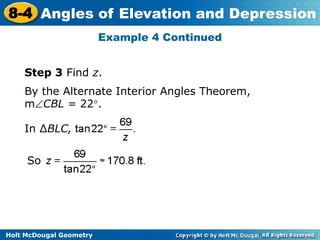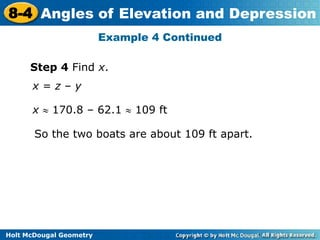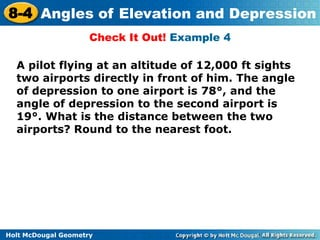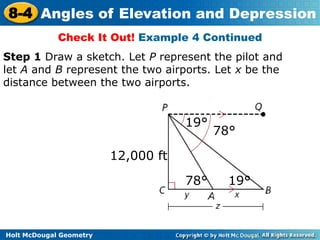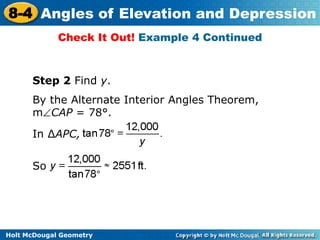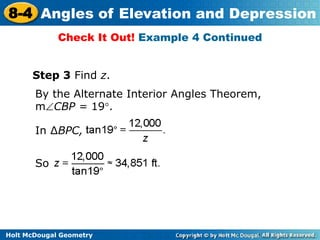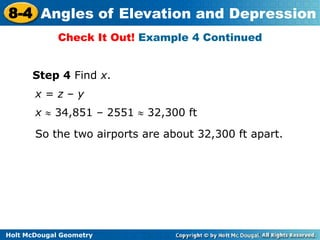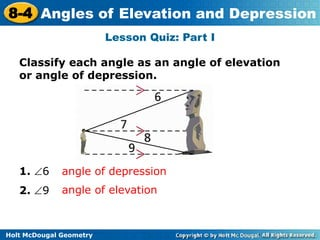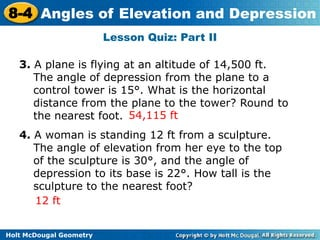1 sur 27
Publicité

### Ao dand aoe

1. GT Drill 3/22/13 • Turn in CW on the bookshelf from yesterday by the time the bell rings. • NO drill Today • Quiz/Test on Trig Thursday • Graded CW and Review next week.
2. 8-4 Angles of Elevation and Depression Objective Solve problems involving angles of elevation and angles of depression. Holt McDougal Geometry
3. 8-4 Angles of Elevation and Depression Vocabulary angle of elevation angle of depression Holt McDougal Geometry
4. 8-4 Angles of Elevation and Depression An angle of elevation is the angle formed by a horizontal line and a line of sight to a point above the line. In the diagram, 1 is the angle of elevation from the tower T to the plane P. An angle of depression is the angle formed by a horizontal line and a line of sight to a point below the line. 2 is the angle of depression from the plane to the tower. Holt McDougal Geometry
5. 8-4 Angles of Elevation and Depression Since horizontal lines are parallel, 1 2 by the Alternate Interior Angles Theorem. Therefore the angle of elevation from one point is congruent to the angle of depression from the other point. Holt McDougal Geometry
6. 8-4 Angles of Elevation and Depression Example 1A: Classifying Angles of Elevation and Depression Classify each angle as an angle of elevation or an angle of depression. 1 1 is formed by a horizontal line and a line of sight to a point below the line. It is an angle of depression. Holt McDougal Geometry
7. 8-4 Angles of Elevation and Depression Example 1B: Classifying Angles of Elevation and Depression Classify each angle as an angle of elevation or an angle of depression. 4 4 is formed by a horizontal line and a line of sight to a point above the line. It is an angle of elevation. Holt McDougal Geometry
8. 8-4 Angles of Elevation and Depression Check It Out! Example 1 Use the diagram above to classify each angle as an angle of elevation or angle of depression. 1a. 5 5 is formed by a horizontal line and a line of sight to a point below the line. It is an angle of depression. 1b. 6 6 is formed by a horizontal line and a line of sight to a point above the line. It is an angle of elevation. Holt McDougal Geometry
9. 8-4 Angles of Elevation and Depression Example 2: Finding Distance by Using Angle of Elevation The Seattle Space Needle casts a 67- meter shadow. If the angle of elevation from the tip of the shadow to the top of the Space Needle is 70º, how tall is the Space Needle? Round to the nearest meter. Draw a sketch to represent the given information. Let A represent the tip of the shadow, and let B represent the top of the Space Needle. Let y be the height of the Space Needle. Holt McDougal Geometry
10. 8-4 Angles of Elevation and Depression Example 2 Continued You are given the side adjacent to A, and y is the side opposite A. So write a tangent ratio. y = 67 tan 70° Multiply both sides by 67. y 184 m Simplify the expression. Holt McDougal Geometry
11. 8-4 Angles of Elevation and Depression Check It Out! Example 2 What if…? Suppose the plane is at an altitude of 3500 ft and the angle of elevation from the airport to the plane is 29°. What is the horizontal distance between the plane and the airport? Round to the nearest foot. You are given the side opposite A, and x is the side adjacent to A. So write a tangent ratio. Multiply both sides by x and divide by tan 29 . x 6314 ft Simplify the expression. 3500 ft 29° Holt McDougal Geometry
12. 8-4 Angles of Elevation and Depression Example 3: Finding Distance by Using Angle of Depression An ice climber stands at the edge of a crevasse that is 115 ft wide. The angle of depression from the edge where she stands to the bottom of the opposite side is 52º. How deep is the crevasse at this point? Round to the nearest foot. Holt McDougal Geometry
13. 8-4 Angles of Elevation and Depression Example 3 Continued Draw a sketch to represent the given information. Let C represent the ice climber and let B represent the bottom of the opposite side of the crevasse. Let y be the depth of the crevasse. Holt McDougal Geometry
14. 8-4 Angles of Elevation and Depression Example 3 Continued By the Alternate Interior Angles Theorem, m B = 52°. Write a tangent ratio. y = 115 tan 52° Multiply both sides by 115. y 147 ft Simplify the expression. Holt McDougal Geometry
15. 8-4 Angles of Elevation and Depression Check It Out! Example 3 What if…? Suppose the ranger sees another fire and the angle of depression to the fire is 3°. What is the horizontal distance to this fire? Round to the nearest foot. 3° By the Alternate Interior Angles Theorem, m F = 3°. Write a tangent ratio. Multiply both sides by x and divide by tan 3 . x 1717 ft Simplify the expression. Holt McDougal Geometry
16. 8-4 Angles of Elevation and Depression Example 4: Shipping Application An observer in a lighthouse is 69 ft above the water. He sights two boats in the water directly in front of him. The angle of depression to the nearest boat is 48º. The angle of depression to the other boat is 22º. What is the distance between the two boats? Round to the nearest foot. Holt McDougal Geometry
17. 8-4 Angles of Elevation and Depression Example 4 Application Step 1 Draw a sketch. Let L represent the observer in the lighthouse and let A and B represent the two boats. Let x be the distance between the two boats. Holt McDougal Geometry
18. 8-4 Angles of Elevation and Depression Example 4 Continued Step 2 Find y. By the Alternate Interior Angles Theorem, m CAL = 58°. In ∆ALC, . So Holt McDougal Geometry
19. 8-4 Angles of Elevation and Depression Example 4 Continued Step 3 Find z. By the Alternate Interior Angles Theorem, m CBL = 22°. In ∆BLC, So Holt McDougal Geometry
20. 8-4 Angles of Elevation and Depression Example 4 Continued Step 4 Find x. x=z–y x 170.8 – 62.1 109 ft So the two boats are about 109 ft apart. Holt McDougal Geometry
21. 8-4 Angles of Elevation and Depression Check It Out! Example 4 A pilot flying at an altitude of 12,000 ft sights two airports directly in front of him. The angle of depression to one airport is 78°, and the angle of depression to the second airport is 19°. What is the distance between the two airports? Round to the nearest foot. Holt McDougal Geometry
22. 8-4 Angles of Elevation and Depression Check It Out! Example 4 Continued Step 1 Draw a sketch. Let P represent the pilot and let A and B represent the two airports. Let x be the distance between the two airports. 19° 78° 12,000 ft 78° 19° Holt McDougal Geometry
23. 8-4 Angles of Elevation and Depression Check It Out! Example 4 Continued Step 2 Find y. By the Alternate Interior Angles Theorem, m CAP = 78°. In ∆APC, So Holt McDougal Geometry
24. 8-4 Angles of Elevation and Depression Check It Out! Example 4 Continued Step 3 Find z. By the Alternate Interior Angles Theorem, m CBP = 19°. In ∆BPC, So Holt McDougal Geometry
25. 8-4 Angles of Elevation and Depression Check It Out! Example 4 Continued Step 4 Find x. x=z–y x 34,851 – 2551 32,300 ft So the two airports are about 32,300 ft apart. Holt McDougal Geometry
26. 8-4 Angles of Elevation and Depression Lesson Quiz: Part I Classify each angle as an angle of elevation or angle of depression. 1. 6 angle of depression 2. 9 angle of elevation Holt McDougal Geometry
27. 8-4 Angles of Elevation and Depression Lesson Quiz: Part II 3. A plane is flying at an altitude of 14,500 ft. The angle of depression from the plane to a control tower is 15°. What is the horizontal distance from the plane to the tower? Round to the nearest foot. 54,115 ft 4. A woman is standing 12 ft from a sculpture. The angle of elevation from her eye to the top of the sculpture is 30°, and the angle of depression to its base is 22°. How tall is the sculpture to the nearest foot? 12 ft Holt McDougal Geometry
Publicité